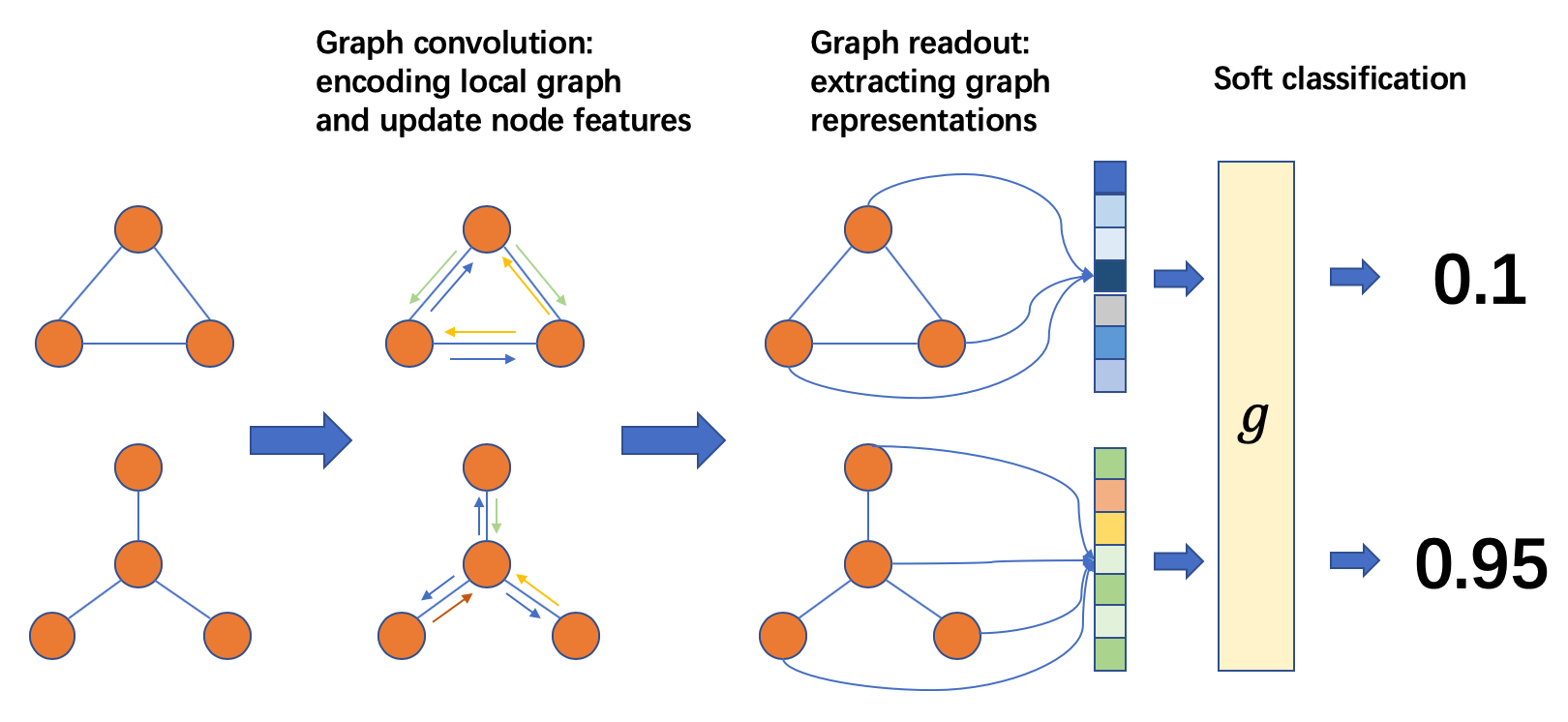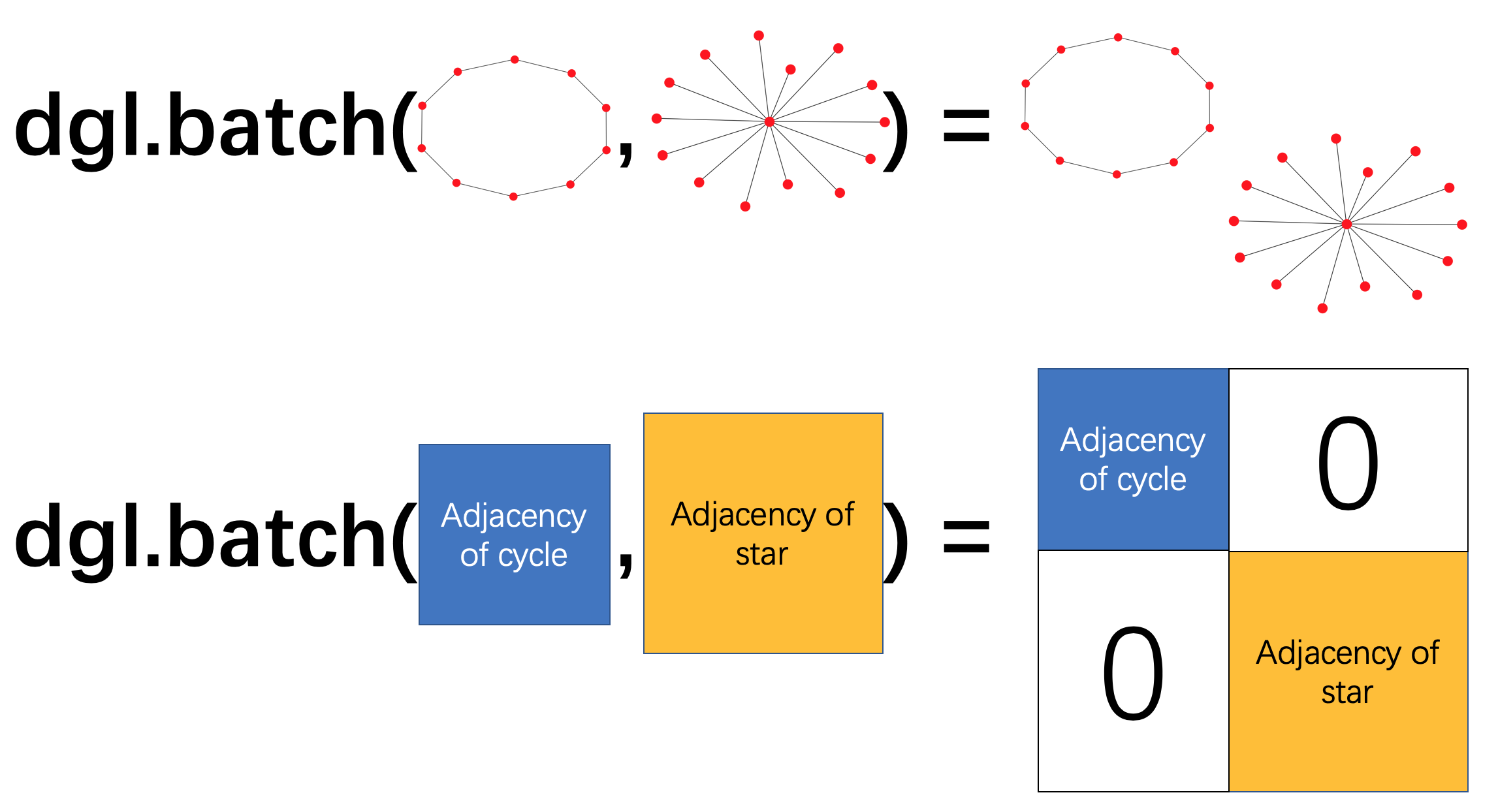# 5.4 整图分类¶

(English Version)

## 概述¶• 准备一个批次的图；

• 在这个批次的图上进行消息传递以更新节点或边的特征；

• 将一张图里的节点或边特征聚合成整张图的图表示；

• 根据任务设计分类层。

### 批次的图¶### 图读出¶

$h_g = \frac{1}{|\mathcal{V}|}\sum_{v\in \mathcal{V}}h_v$

DGL内置了常见的图读出函数，例如 dgl.readout_nodes() 就实现了上述的平均值读出计算。

## 编写神经网络模型¶

### 批次化图上的计算¶

import dgl
import torch

g1 = dgl.graph(([0, 1], [1, 0]))
g1.ndata['h'] = torch.tensor([1., 2.])
g2 = dgl.graph(([0, 1], [1, 2]))
g2.ndata['h'] = torch.tensor([1., 2., 3.])

# tensor([3.])  # 1 + 2

bg = dgl.batch([g1, g2])
# tensor([3., 6.])  # [1 + 2, 1 + 2 + 3]


bg.ndata['h']
# tensor([1., 2., 1., 2., 3.])


### 模型定义¶

import dgl.nn.pytorch as dglnn
import torch.nn as nn

class Classifier(nn.Module):
def __init__(self, in_dim, hidden_dim, n_classes):
super(Classifier, self).__init__()
self.conv1 = dglnn.GraphConv(in_dim, hidden_dim)
self.conv2 = dglnn.GraphConv(hidden_dim, hidden_dim)
self.classify = nn.Linear(hidden_dim, n_classes)

def forward(self, g, h):
# 应用图卷积和激活函数
h = F.relu(self.conv1(g, h))
h = F.relu(self.conv2(g, h))
with g.local_scope():
g.ndata['h'] = h
# 使用平均读出计算图表示
hg = dgl.mean_nodes(g, 'h')
return self.classify(hg)


## 模型的训练¶

### 数据加载¶

import dgl.data
dataset = dgl.data.GINDataset('MUTAG', False)


from dgl.dataloading import GraphDataLoader
dataset,
batch_size=1024,
drop_last=False,
shuffle=True)


import torch.nn.functional as F

# 这仅是个例子，特征尺寸是7
model = Classifier(7, 20, 5)
for epoch in range(20):
feats = batched_graph.ndata['attr']
logits = model(batched_graph, feats)
loss = F.cross_entropy(logits, labels)
loss.backward()
opt.step()


DGL实现了一个整图分类的样例： DGL的GIN样例。 模型训练的代码请参考位于 main.py 源文件中的 train 函数。 模型实现位于 gin.py ， 其中使用了更多的模块组件，例如使用 dgl.nn.pytorch.GINConv 模块作为图卷积层(DGL同样支持它在MXNet和TensorFlow后端里的实现)、批量归一化等。

## 异构图上的整图分类模型的训练¶

class RGCN(nn.Module):
def __init__(self, in_feats, hid_feats, out_feats, rel_names):
super().__init__()

self.conv1 = dglnn.HeteroGraphConv({
rel: dglnn.GraphConv(in_feats, hid_feats)
for rel in rel_names}, aggregate='sum')
self.conv2 = dglnn.HeteroGraphConv({
rel: dglnn.GraphConv(hid_feats, out_feats)
for rel in rel_names}, aggregate='sum')

def forward(self, graph, inputs):
# inputs是节点的特征
h = self.conv1(graph, inputs)
h = {k: F.relu(v) for k, v in h.items()}
h = self.conv2(graph, h)
return h

class HeteroClassifier(nn.Module):
def __init__(self, in_dim, hidden_dim, n_classes, rel_names):
super().__init__()

self.rgcn = RGCN(in_dim, hidden_dim, hidden_dim, rel_names)
self.classify = nn.Linear(hidden_dim, n_classes)

def forward(self, g):
h = g.ndata['feat']
h = self.rgcn(g, h)
with g.local_scope():
g.ndata['h'] = h
# 通过平均读出值来计算单图的表征
hg = 0
for ntype in g.ntypes:
hg = hg + dgl.mean_nodes(g, 'h', ntype=ntype)
return self.classify(hg)


# etypes是一个列表，元素是字符串类型的边类型
model = HeteroClassifier(10, 20, 5, etypes)